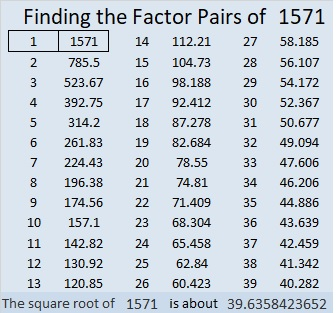# 1571 Candy Cane

### Today’s Puzzle:

The shepherds’ crooks from that first Christmas night have become the sweet candy canes we often see on today’s Christmas trees. Can you find the factors from 1 to 12 that will make this mystery level puzzle function like a multiplication table? Remember to use logic to find the factors.### Factors of 1571:

• 1571 is a prime number.
• Prime factorization: 1571 is prime.
• 1571 has no exponents greater than 1 in its prime factorization, so √1571 cannot be simplified.
• The exponent in the prime factorization is 1. Adding one to that exponent we get (1 + 1) = 2. Therefore 1571 has exactly 2 factors.
• The factors of 1571 are outlined with their factor pair partners in the graphic below.How do we know that 1571 is a prime number? If 1571 were not a prime number, then it would be divisible by at least one prime number less than or equal to √1571. Since 1571 cannot be divided evenly by 2, 3, 5, 7, 11, 13, 17, 19, 23, 29, 31, or 37, we know that 1571 is a prime number.

### More about the Number 1571:

1571 is the sum of two consecutive numbers:
785 + 786 = 1571.

1571 is also the difference of two squares:
786² – 785² = 1571.

Do you see the relationship between those two facts?